1.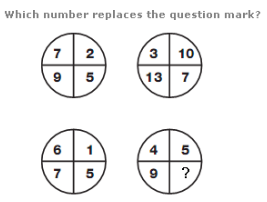A. 1 B. 2 C. 3 D. 4

Explanation: :

In each circle, the number in the lower left segment equals the sum of the numbers in the top two segments, and the number in the lower right circle equals the difference between the numbers in the top two segments.

2.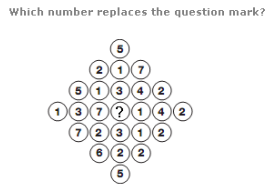A. 2 B. 3 C. 4 D. 5

Explanation: :

Working in rows, the sum of numbers in each row follows the sequence 5, 10, 15, 20, 15, 10, 5.

3.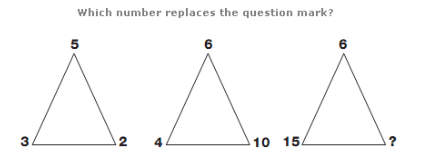A. 19 B. 16 C. 12 D. 9

Explanation: :

Working from left to right, the sum of the two smaller numbers on each triangle equals the third number.

4.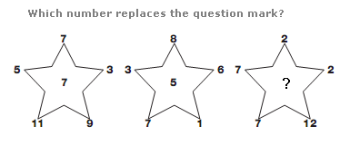A. 8 B. 13 C. 15 D. 6

Explanation: :

The number in the centre of each star equals the average of the surrounding 5 numbers.

5.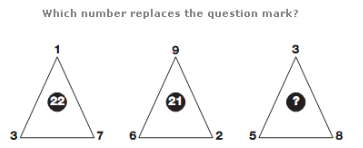A. 45 B. 34 C. 43 D. 54